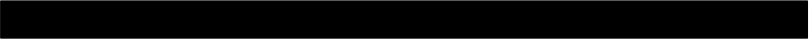We will calculate these probabilities using Bayes' Theorem, which is shown in the box below:

p(A|B)

=

p(B|A)

p(A)

p(B)This is the posterior

This is the likelihood

This is the prior

This is the z

We can use this equation to rewrite the probabilities that we want to calculate. For example, type what p(black and blue | image) would become when transformed using Bayes' Theorem:

Remember that all we care about is whether p(black and blue | image) or p(white and gold | image) is greater. We don't actually care what the exact values of these probabilities are, as long as we can tell which one is larger. Therefore we can ignore the denominator and just write:

You should read these equations as "the probability of black and blue given the image is proportional to the probability of the image given black and blue times the probability of black and blue."